# ACT Maths Practice Questions with Detailed Solutions - sample 1

Detailed solutions and explanations to 60 Maths questions are presented. The questions are those in ACT sample 1.

1. 4 x 10-5 =
Solution
Rewrite 4 x 10-5 as
4 / 10-5 = 4 / 100,000 = 0.00004

2. Which of the following is equivalent to (x)(x)(x)(x3), for all x?
Solution
Rewrite (x)(x)(x)(x3) as
(x)(x)(x)(x3) = (x)3 (x3) = x 6

3. There are 15 balls in a box: 8 balls are green, 4 are blue and 3 are white. Then 1 green and 1 blue balls are taken from the box and put away. What is the probability that a blue ball is selected at random from the box?
Solution
If 1 green and 1 blue ball are taken from the box, then there are 7 green, 3 blue and 3 white balls, a total of 13 balls. If one ball is selected at random, the probability that it is blue is given by
3 / 13

4. The length of a rectangle is 3 times its width. If the width of the rectangle is 5 inches, what is the rectangle's area, in square inches?
Solution
If the width is 5 in and the length is 3 times the width, then the length is
3 * 5 = 15 in
The area is given by
5 * 15 = 75 in2

5. For all x >2, (2x2 + 2x - 12) / (x - 2) simplifies to
Solution
We first factor 2x2 + 2x - 12 as follows
2x2 + 2x - 12 = 2(x2 + x - 6)
= 2(x + 3)(x - 2)
We simplify as follows
(2x2 + 2x - 12) / (x - 2) = [ 2(x + 3)(x - 2) ] / (x - 2) = 2(x + 3)

6. If the hypotenuse of a right triangle is 10 inches long and one of its legs is 5 inches long, how long is the other leg?
Solution
Let x be the length of second leg and apply Pythagora's theorem as follows
102 = 52 + x2
Solve for x
x = √(100 - 25) = √75 = 5 √3

7. In the standard (x,y) coordinate plane, the graph of (x + 3)2 + (y + 5)2 = 16 is a circle. What is the circumference of the circle, expressed in coordinate units?
Solution
rewrite the given equation as follows
(x + 3)2 + (y + 5)2 = 42
The radius of the circle is 4 and therefore the circumference is given by
2 * 4 * π = 8 π

8. How many solutions are there to the equation x2 - 7 = 0?
Solution
Rewrite the given equation as follows
x2 = 7
The above equation has two solutions + √7 and - √7

9. A circle with center (4,-5) is tangent to the y-axis in the standard (x,y) coordinate plane. What is the radius of this circle?
Solution
The radius is given by the distance between the center of the circle (4 , - 5) and the y axis which is 4

10. Angle A is an acute angle and sin(A) = 11/14. What is the value of cos(A)?
Solution
sin(A) and cos(A) are related by
sin(A)2 + cos(A)2 = 1
Substitute sin(A) by 11/14 in the above equation
(11/14)2 + cos(A)2 = 1
Solve for cos(A) and select the positive value since A is an acute angle
cos(A) = √(1 - 121 / 196)
= √[ (196 - 121) / 196 ]
= √(196 - 121) / 14 = √75 / 14 = 5 √3 / 14

11. What are the values of a and b, if any, where - a|b + 4| > 0?
Solution
For - a |b + 4| - a must be positive and |b + 4| must also be positive. Which means a must be negative and b + 4 not equal to 0 or b not equal to - 4.
a < 0 and b not equal to - 4.

12. In a shipment of televisions, 1/50 of the televisions are defective. What is the ratio of defective to nondefective televisions?
Solution
If 1/50 then
1 - 1/50 = 49 / 50 are not defective
So if x is the total number of TV sets, then (1/50)x are defective and (49/5)x are not defective. Hence the ratio of defective to non defective is given by
x(1 / 50) / x(49 / 50) = (1/50)(50/49) = 1/49

13. Which of the following is divisible (with no remainder) by 4?
Solution
A whole number is divisible by 4 if the number formed by the last two digits is divisible by 4. The nubers formed by the the last two digits of the given numbers are: 33, 56, 69, 17 and 95. The only one that is divisible by 4 is 56 and therefore 510056 is the only number in the list that is divisible by 4.

14. A particle travels 1 x 106 meters per second in a straight line for 5 x 10-6 seconds. How many meters has it traveled?
Solution
We are given the speed 1 x 106 m/s and the time 5 x 10-6 seconds. The question is to find how many meters meaning the distance d. Hence d is given by
d = ( 1 x 106 m/s ) *( 5 x 10-6 s )
= 1 x 106 x 5 x 10-6
= 5 106 + (-6)
= 5 100 = 5 meters

15. The lengths of sides AB and AC in the triangle below are equal. What is the measure of angle ∠ A?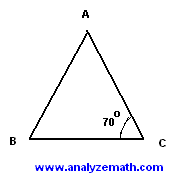.

Solution
Since the lengths of sides AB and AC are equal, then the triangle is isosceles and therefore angles B and C are equal in size. Hence angle A is given by
180 - (70o + 70o) = 40o

16. In the figure below ABC is an equilateral triangle. AH is perpendicular to BC and has a length of 2√3 inches. What is the area, in square inches, of triangle Δ ABC.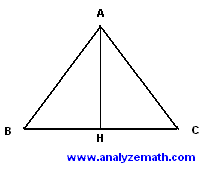.

Solution
Let x be the length of the side of the equilateral triangle. Since BH is perpendicular to BC, then by Pythagora's theorem (applied to triangle ABH) we can write
x2 = (x/2)2 + (2√3)2
Simlify the above equation
x2 = x2 / 4 + (4)(3)
4 x2 = x2 + (16)(3)
3 x2 = (16)(3)
x2 = 16
Solve to find x = 4
The area A of the triangle is given by
A = (1/2)AH*BC = (1/2) * 2 √3 * 4 = 4 √3

17. If 8y = 3x - 11, then x =

Solution
We are asked to solve for x hence the need to isolate x. add 11 to both sides of the equation and simplify
8y + 11 = 3x - 11 + 11
8y + 11 = 3x
Divide both sides of the equation by 3 in order to find x as follows
(8y + 11) / 3 = x

18. Which of the statements describes the solution set for -2(x + 8) = -2x + 20?
A) x = - 2 only
B) x = 0 only
C) x = 20 only
D) There are no solution for this equation.
E) All real numbers are solutions of this equation.
Solution
Expand the term on the left hand term of the equation
-2x - 16 = - 2x + 20
Add -2x to both sides of the equation
-2x - 16 + 2x = - 2x + 20 + 2x
-16 = 20
The last statement is always false, hence the given equation has no solution.

19. When graphed in the (x,y) coordinate plane, at what point do the lines 2x + 3y = 5 and x = -2 intersect?
Solution
To find the point of intersection of two lines, we need to solve the system of equations made up of the equations of the lines. We need to solve the following system of equations.
2x + 3y = 5 and x = - 2
Substitute x by -2 in the equation 2x + 3y = 5 and solve for y.
2(-2) + 3y = 5
3y = 9
y = 3
The two lines intersect at (-2 , 3).

20. The area of a trapezoid is 0.5h(b1 + b2), where h is the altitude, and b1 and b2 are the lengths of the parallel bases. If a trapezoid has an alitude of 15 inches, an area of 105 square inches, and one of the bases 22 inches, what is the perimeter, in inches, of the trapezoid?
Solution
Given the area, h and b1, let us find b2.
105 = 0.5*15*(22 + b2)
b2 = 105 / (0.5*15) - 22 = 14 - 22
The base of trapezoid cannot be negative and therefore no trapezoid exists with the given dimensions.

21. If you drove at average speed of 66 miles per hour, what distance, in miles, did you drive in 99 minutes?
Solution
We first convert the speed into miles per minute.
66 / 60 = 1.2 miles per minute
We now use the speed and the time to find the distance.
1.2 (miles/minute) * 99 minutes = 108.9 miles

22. If x and y are any real numbers such that 0 < x < 2 < y , which of these must be true?
A) x < (xy)/2 < y
B) 0 < xy < 2x
C) x < xy < 2
D) 0 < xy < 2
E) xy < y
Solution
Since both x and y are positive, then the inequality x < 2 < y may be written as.
1 / x > 1 / 2 > 1 / y
Multiply all terms of the above inequality by x y and simplify to obtain.
y > x y / 2 > x
The above inequality may also be written as.
x < x y / 2 < y , which correspond to the answer in A) above.
Using the values x = 1.8 and y = 2.1, it can easily be shown that B) C) D) and E) above are not true.

23. In the right triangle ABC below, what is the cosine of angle A?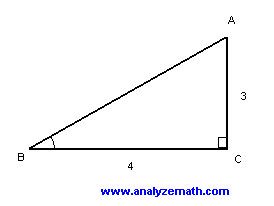.

Solution
We first find the hypotenuse BA and use it to find cos(A).
BA = √(32 + 42) = 5
cos(A) = adajacent side / hypotenuse = 3/5

24. The graph of y = 2 / (x - 3) is shown below.

Among the following, which is the best possible graphical representation of y = -2 / |x - 3|.

Solution
|x - 3| is always positive or zero. Hence - 1 / |x - 3| is always negative except at x = 3 where it is not defined. The graph in C is all below the x axis and is therefore the closet to the graph of y = -2 / |x - 3|

25. What is the slope of the line
4x = -3y + 8

Solution
We first need to write the given equation in slope intercept form y = m x + b and then identify the slope and its value.
4x = -3 y + 8 given
-3 y = 4 x - 8
y = -(4 / 3)x + 8 / 3
The slope is the coefficient of and is equal to - 4 / 3.

26. In the figure below, B is on AD, ∠ BAC and ∠ ACB measure 26� and 131� respectively. What is the measure of ∠CBD?.

Solution
Let us first find angle ABC using the sum of the angles in triangle ABC.
size of angle ABC = 180� - (26� + 131�) = 23�
Angles ABC and CBD are supplementary. Hence
size of angle CBD = 180� - 23� = 157�

27. Which of the following is equal to √45
Solution
√45 = √(9 * 5) = √9 √5 = 3 √5

28. What is the smallest value of x that satisfies the equation
x(x + 4) = -3

Solution
Expand and write the given equation with the right side equal to 0.
x 2 + 4x + 3 = 0
The left side of the above equation may be factored as follows.
(x + 3)(x + 1) = 0
The two solutions are.
x = - 3 and x = - 1 and the smallest is - 3

29. A group of 7 friends are having lunch together. Each person eats at least 3/4 of a pizza. What is the smallest number of whole pizzas needed for lunch?
Solution
If each person eats at least 3/4 of a pizza, then the 7 will eat at least.
7 * (3 / 4) = 21 / 4 = 5 + 1/4
At least 6 pizzas are needed for lunch.

30. All axes in the graphs below have the same scales. Which one of the graphs is closet to the graph of y = -2x + 2?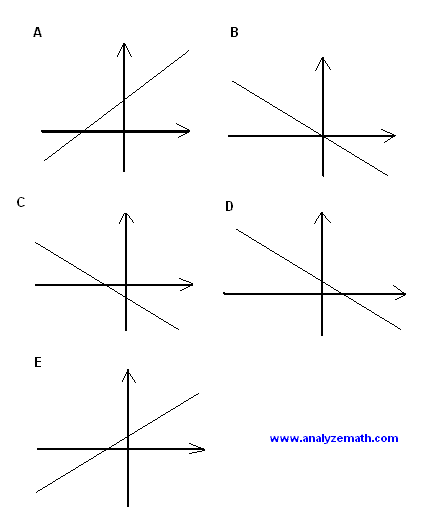.

Solution
let us find the x and y intercepts of the line whose equation is given.
x intercept: y = 0 , 0 = -2 x + 2 , x = 1 , (1 , 0)
y intercept: x = 0 , y = 2 , x = 1 , (0 , 2)
Using the x and y intercepts the closet graph to the graph of y = -2x + 2 is the graph D).

31. The measures of angles A, B and C of a triangle are in the ratio 3:4:5. What is the measure, in degrees, of the largest angle?
Solution
Let the sizes of angles A, B and C be written as follows
A = 3 x, B = 4 x and C = 5 x , where x is a common factor.
The sum of all three angles is equal to 180�. hence
3 x + 4 x + 5 x = 180�
Solve the above equation for x
x = 15
The size of the largest angle is equal to
5 x = 5 * 15 = 75�

32. There are n students in a school. If r% among the students are 12 years or younger, which of the following expressions represents the number of students who are older than 12?
Solution
A student may either be 12 years or younger or older than 12. So if r% are are 12 years or younger then (100 - r)% are older than 12. Hence the number of students who are older than 12 is given by
n * (100 - r)% = n(100 - r) / 100

33. If x + 4y = 5 and 5x + 6y = 7, then 3x + 5y = ?
Solution
Add the left terms and right terms of the two equation to obtain a new equation
x + 5x + 4y + 6y = 5 + 7
Group and simplify
6x + 10y = 12
Divide all terms of the above equation by 2 to obtain a new equation
3x + 5y = 6

34. Which integer is nearest to √2100 / √7?
√2100 / √7 = √(2100 / 7) = √(300) = 17.32
The nearest integer is 17

35. For all real numbers x, the minimum value of 1 + 2 cos(4x) is
Solution
The range of values of cos(4x) is as follows
- 1 ≤ cos(4x) ≤ 1
Multiply all terms of the above inequality by 2 to obtain
- 2 ≤ 2 cos(4x) ≤ 2
Add +1 to all terms of the inequality and simplify
- 2 + 1 ≤ 2 cos(4x) + 1 ≤ 2 + 1
-1 ≤ 2 cos(4x) + 1 ≤ 3
The minimum value of 1 + 2 cos(4x) is - 1

36. What is the largest possible product for 2 odd integers whose sum is equal to 32?
Solution
The two odd numbers may be written as follows
2 n + 1 and 2 k + 1 where n and k are positive integers
The sum of the two numbers is equal to 32
2n + 1 + 2k + 1 = 32
The above gives
n + k = 15 or k = 15 - n
The product P of the two odd integers is given by
P = (2n + 1)(2k + 1)
= (2n + 1)(2(15 - n) + 1)
= - 4 n 2 + 60 n + 31
P = - 4 (n - 15/2) 2 + 4 (15 / 2) 2 + 31
The product P is maximum for n = 15 / 2 = 7.5. However n must be an integer and therefore P is maximum for integer values of n equal to 7 or 8 and for both these values P = 255
Check:
1) n = 7 , k = 15 - n = 8 , 2n + 1 = 15 and 2k + 1 = 17, product = 255, sum = 32.
2) n = 8 , k = 15 - n = 7 , 2n + 1 = 17 and 2k + 1 = 15 , product = 255 , sum = 32.

37. If (a + b)2 = 25 and (a - b)2 = 45, then a2 + b2 = ?
Solution
Expand the right hand side of each equation given above
a2 + b2 + 2ab = 25 and a2 + b2 - 2ab = 45
Add the left hand term and the right hand terms of the two equations and simplify
2 a2 + 2 b2 = 25 + 45 = 70
Divide by 2 all terms in the above equation to obtain
a2 + b2 = 35

38. If a = 3, then 2 / (1/7 + 1/a) = ?
Solution
For a = 3
2 / (1/7 + 1/a) = 2 / (1/7 + 1/3) = 2 / [ (3 + 7) / 21 ] = 21 / 5

39. A company makes a profit equal to 25% of its sales. The profit is shared equally among the 4 owners of the company. If the company generates sales of $5,000,000, how much money does each one of the owners get? Solution The profit made on sales of$5,000,000 is given by
25% * $5,000,000 =$ 1,250,000
The profit made by each one of the 4 owners is given by
$1,250,000 / 4 =$ 312,500

40. If the expression x3 + 2hx - 2 is equal to 6 when x = -2, what is the value of h?
Solution
x3 + 2hx - 2 = 6 for x = - 2. Hence
(-2)3 + 2h(-2) - 2 = 6
Solve the above for h
h = - 4

41. If -3/(a - 3) = 3/(a + 2), then a = ?
Solution
Multiply all terms of the given equation by (a - 3)(a + 2), simplify and solve
(a - 3)(a + 2)[ -3/(a - 3) ] = (a - 3)(a + 2) [ 3/(a + 2) ]
- 3 (a + 2) = 3 (a - 3)
a = 1/2

42. For what negative value of k, the system of equations below has no solutions?
2x + ky = 5
kx + (k + 4)y = 7
Solution
For the above system of equations to have no solutions, the determinant of the coefficient matrix of the system (shown below) must be zero.
 2 k k k+4

2(k + 4) - k2 = 0
Expand and solve for k
- k 2 + 2k + 8 = 0
solutions to above quadratic equation: k = -2 and k = 4
The negative value of k for the above system has no solutions is - 2.

43. The two legs of a right triangle measure 6 and 8 inches respectively. What is the area of the circle that contains all 3 vertices of the triangle?
Solution
The hypotenuse of right triangle inscribed in a circle is equal in size to the diamter of the circle. We use Pythagora's theorem to find the size of the hypotenuse h.
h2 = 62 + 82 = 100
h = 10
Diameter = h = 10 and radius = 5
Area of the circle = Pi 52 = 25 Pi

44. X and Y are acute angles such that tan Y = cot X. What is the sum, in degrees, of the measures of the angles X and Y?
Solution
tan Y = cot X gives.
tan Y = 1 / tan X which gives tan Y * tan X = 1
we now use the tangent formula for a sum of angles Y and X.
tan(X+Y) = (tan X + tan Y) / (1 - tan X * tan Y)

Since tan Y * tan X = 1, tan(X+Y) is undefined and therefore X + Y = 90� .

45. The measures of the sides of the right triangle below are in inches. What is the value of x?.

Solution
tan(60�) = 1 / x
Solve for x
x = 1 / tan(60�) = 1 / √3

46. Which of the graphs below is the solution set of -3x ≤ 12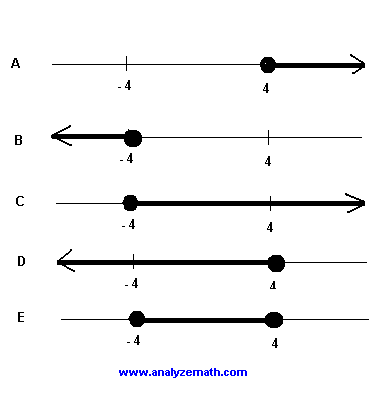.

Solution
Divide both sides of the given inequality by - 3 and reverse the inequality symbol
- 3x / - 3 ≤ 12 / - 3
x ≤ -4
The above inequality corresponds to the graph in C).

47. Which of the lines below is not parallel to the line 6x - 2y = 10?
A) 3x - y = 7
B) -6x + 2y = 20
C) 3x + y = 7
D) 6x - 2y = 5
E) x - y/3 = 9
Solution
Let us find the slope of the given line
6x - 2y = 10 , solve for y: y = 3x - 5 , slope = 3
One can find the slopes of all lines in A) B) C) D) and E). But a quick examinations of all these equations shows that the slope in line C) is not equal to 3 and hence not parallel to the line 6x - 2y = 10. Let us find the slope of the line in C)
3x + y = 7 , solve for y: y = - 3x + 7 , slope - 3, this line is NOT parallel to the given line.

48. For what value of k the equation below has no solution?
2x + 3 = x - 2kx - 5

Solution
Let us solve for x the given equation
2x + 3 = x - 2kx - 5
x + 2 k x = - 8
x(1 + 2 k) = - 8
x = - 8 / (1 + 2 k)
The solution x is a real number and therefore the given equation has a solution except when 1 + 2 k = 0 since a division by 0 is not allowed. Hence the given equation has no solution if
1 + 2 k = 0 or k = - 1/2

49. |5 - 2| - |6 - 9| = ?

Solution
|5 - 2| - |6 - 9| = | 3 | - | -3 | = 3 - 3 = 0

50. What is the average of 7/8 and 3/4?
Solution
average of 7/8 and 3/4 = (7/8 + 3/4) / 2 = (7/8 + 6/8) / 2 = (13 / 8) / 2 = 13 / 16

51. A solution is made of water and pure acid. If 75% of the solution is water, how many litters of pure acid are in 20 litters of this solution?
Solution
If 75% of the solution is water then
100% - 75% = 25% is acid.
If the solution is 20 litters, then
25% of 20 = 25% * 20 = 5 litters are pure acid.

52. The total surface area of all six faces of the rectangular box below is equal to 128 square inches. What is x in inches?.

Solution
The total area of the front and back faces is given by
2(4x)
The total area of the left and right faces is given by
2(4x)
The total area of the top and bottom faces is given by
2(16)
The total area of all 6 faces is given by
2(4x) + 2(4x) + 2(16) = 16x + 32 = 128
Solve the above for x
x = 6 inches

53. The diagonal of a square has a measure of 12 inches. What is the perimeter, in inches, of this square.
Solution
Let x be the side of a square. Use Pythagora's theorem to write a relationship between two sides and the diagonal.
x 2 + x 2 = 12 2
Solve the above equation to find the side x.
x = 12 / √2 = 6 √2
The perimeter is given by.
4x = 24√2

54. The lengths in the rectangle below are in inches. What is the shaded area in square inches?.

Solution
The total area of the rectangle is given by
7*(6 + 4) = 70 square inches
The non shaded part of the rectangle is made up of three triangles whose total area is given by
(1/2)*6*7 + (1/2)*4*4 + (1/2)*3*2 = 32 square inches
The area of the shaded region is equal to the total area of the rectangle minus the total non shaded area. Hence
70 - 32 = 38 square inches

55. In the right triangle ABC, C is a right angle and the measure of angle B is 60�. If BC is 20 inches long, then how long is AC?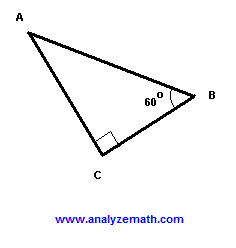.

Solution
Use angle B to write
tan(60�) = AC / BC
Solve for AC
AC = BC tan(60�) = 20 √3

56. In the figure below ABC is a right triangle. ABDE is a square of area 200 square inches and BCGF is a square of 100 square inches. What is the length, in inches, of AC?.

Solution
If the area of ABDE is 200, then
AB = √200 = 10√2 inches
If the area of BCGF is 100, then
BC = √100 = 10 inches
We now use Pythagora's theorem in the right triangle ABC to find AC
AC2 = (10√2)2 + 102
AC = 10√3

57. If x = 2.0001, which of the following expressions has the largest value?
A) 2 / (x + 2)
B) 2 / (x - 2)
C) (x + 2) / 2
D) 2 / x
E) x / 2
Solution
Examining the 5 expressions, the expression in B) has a denominator equal to x - 2 = 2.0001 - 2 = 0.0001. A small denominator leads to a larger value.

58. In the rectangle below, the measure of the length AD is 3 times the measure of the width AB. What is the slope of the line segment BD?.

Solution
Slope of BD
= - CD / AD = - AB / AD = - AB / (3*AB) = - 1 / 3

59. What is the product of the two real solutions of the equation

2x = 3 - x2

Solution
Factor and solve equation 2x = 3 - x2
x2 + 2 x - 3 = 0
(x - 1)(x + 3) = 0
Solutions: x = 1 and x = -3
Product of the solutions = 1*(-3) = -3

60. Which of the following equations corresponds the data in the table?
 x y -20 -1/8 -10 -1/3 0 1/2 5 2/9 20 1/12

A) y = 1 / ( x + 2)
B) y = 2 / ( x + 4)
C) y = (x - 1) / ( x + 2)
D) y = 2 / ( x - 2)
E) y = 1 / ( 2x + 2)
Solution
Using (0 , 1/2) in the given table, it can easily be checked that the equations in C) and D) do not correspond to the data in the table.
C) y = (x - 1) / ( x + 2) : x = 0 gives y = -1/2
D) y = 2 / ( x - 2) : x = 0 gives y = -1
Using (5 , 2/9), we can easily check that equations in A) and E) do not correspond to the data in the table.
A) y = 1 / ( x + 2) : x = 5 gives y = 1/7
E) y = 1 / ( 2x + 2) : x = 5 gives y = 1/12
Equation in B) y = 2 / ( x + 4) corresponds to the data in the table.

More Maths Practice Tests
Free Practice for SAT, ACT Maths tests
Free Practice for GAMT Maths tests
Free Compass Maths tests Practice
Free GRE Quantitative for Practice
Free AP Calculus Questions (AB and BC) with Answers